# The electric field on the x axis due to a point charge fixed at the origin...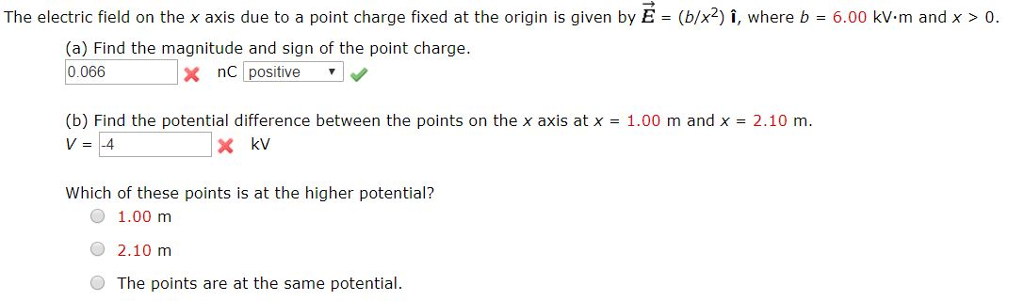The electric field on the x axis due to a point charge fixed at the origin is given by E = (b/x2)I, where b = 6.00 kv. m and x > 0. (a) Find the magnitude and sign of the point charge. 0.066 x nC positive (b) Find the potential difference between the points on the x axis at x V=-4 1.00 m and x 2.10 m. Which of these points is at the higher potential? 74%. O 1.00 m 2.10 m The points are at the same potential.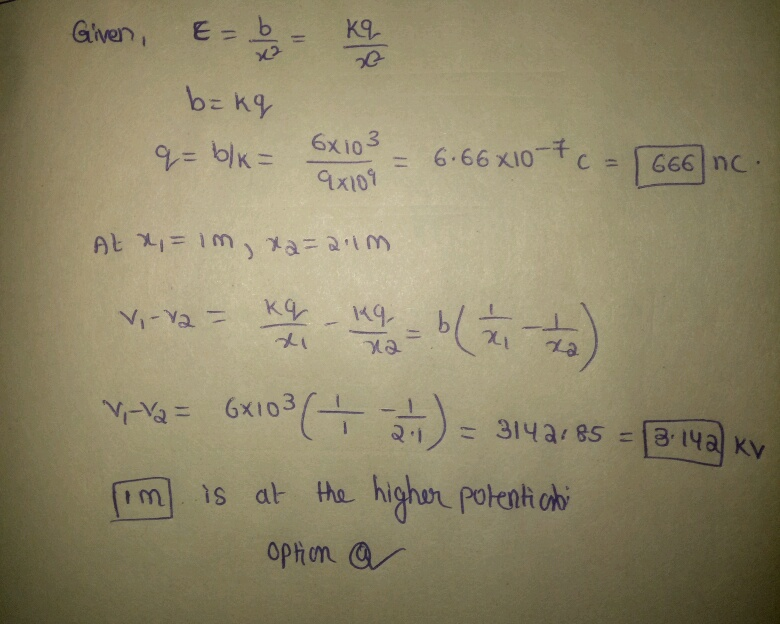#### Earn Coin

Coins can be redeemed for fabulous gifts.

Similar Homework Help Questions
• ### The electric field on the x axis due to a point charge fixed at the origin is given by Earrowbolditalic = (b/x2) ihatbo...

The electric field on the x axis due to a point charge fixed at the origin is given by Earrowbolditalic = (b/x2) ihatbold, where b = 5.00 kV·m and x > 0. (a) Find the magnitude and sign of the point charge. (in nC) (b) Find the potential difference between the points on the x axis at x = 1.50 m and x = 2.10 m.

• ### Problem 1 A -2.80 jIC point charge is fixed at the origin of the x axis...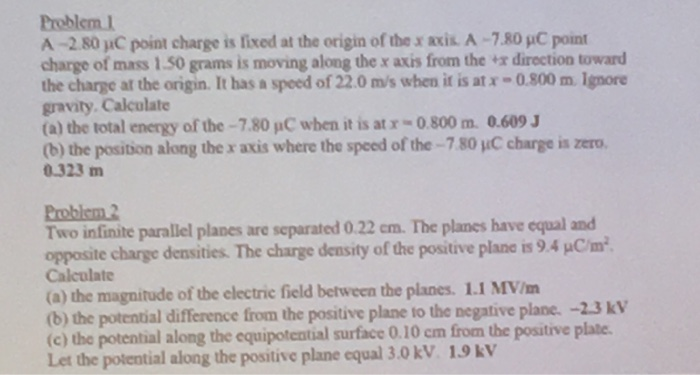Problem 1 A -2.80 jIC point charge is fixed at the origin of the x axis A-7.80 pC point charge of mass 1.50 grams is moving along the x axis from the "x direction toward the charge at the origin. It has a speed of 22.0 m/s when it is at x -0.800 mIgnore gravity. Calculate (a) the total energy of the-7.80 pC when it is at r-0.800 m. 0.609 (b) the position along the x axis where the speed...

• ### Find the electric field at the origin, point O.Two point charges are placed on the x axis. The first charge, q1 = 8.00 nC, is placed a distance 16.0 m from the origin along the positive x axis; the secondcharge, q2 = 6.00 nC, is placed a distance 9.00 m from the origin along the negative x axis.Find the electric field at the origin, point O.=(Give the x and y components of the electric field as an ordered pair. Express your answer in newtons per coulomb to three...

• ### A point charge of -4.00 nC is at the origin, and a second point charge of...

A point charge of -4.00 nC is at the origin, and a second point charge of 6.00 nC is on the x axis at x = 0.810 m . Find the magnitude and direction of the electric field at each of the following points on the x axis. A) x=21.0cm B) 1.30m C) -19.0cm

• ### Two point charges are placed on the x axis. (Figure 1)The first charge, q1 = 8.00...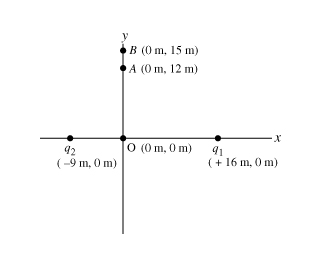Two point charges are placed on the x axis. (Figure 1)The first charge, q1 = 8.00 nC , is placed a distance 16.0 m from the origin along the positive x axis; the second charge, q2 = 6.00 nC , is placed a distance 9.00 m from the origin along the negative x axis. An unknown additional charge q3 is now placed at point B, located at coordinates (0 m, 15.0 m ). Find the magnitude and sign of q3...

• ### Three point charges are arranged along the x axis. Charge q 1 = -4.20 nC is...

Three point charges are arranged along the x axis. Charge q 1 = -4.20 nC is located at x= 0.220 m and charge q 2 = 2.10 nC is at x= -0.330 m . A positive point charge q 3 is located at the origin. 1)What must the value of q3 be for the net force on this point charge to have magnitude 4.10 μN? 2)Where along the x axis can q3 be placed and the net force on it...

• ### A particular charge distribution produces an electric field along the x-axis of the form, a/x2, where...

A particular charge distribution produces an electric field along the x-axis of the form, a/x2, where a is a positive constant. Find the expression for potential difference, on the x-axis, between x = A and x = B. That is, find V (B) – V (A). What charge distribution produced this?

• ### What is the net electric field at the origin, point O?Two point charges are placed on the x axis. The first charge, q1 = 8.00 nC, is placed a distance 16.0 m from the origin along the positive x axis; the secondcharge, q2 = 6.00 nC, is placed a distance 9.00 m from the origin along the negative x axis.Now, assume that charge is negative; .What is the net electricfield at the origin, point O?=(Give the x and y components of the electric field as an ordered pair. Express your...

• ### A point charge at the origin of a coordinate system produces the electric field on the axis at...

A point charge at the origin of a coordinate system produces the electric field E=(36000N/C)x on the x axis at the location x = -0.60m .A)Determine the sign of the chargeB)Determine the magnitude of the charge.Express your answer using two significant figures.

• ### v3. At what distance from a point charge of 4.0 nC does the electric field have...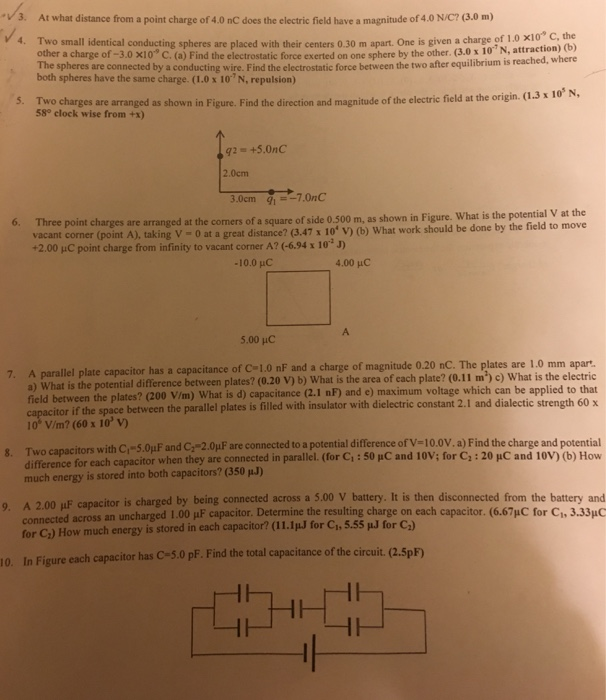v3. At what distance from a point charge of 4.0 nC does the electric field have a magnitude of 4.0 N/C? (3.0 m) 4. Two small identical conducting spheres are placed with their centers 0.30 m apart. One is given a charge of 1 0 x10* c other a charge of -3.0 x10 C. (a) Find the electrostatic force exerted on one sphere by the other. (3.0 x 10"N, attraction) the (b) e spheres are connected by a conducting wire....

Free Homework App